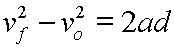# Introduction to the Time Independent Acceleration Equation

This equation relates final velocity, original velocity, constant acceleration, and displacement:The square of the final velocity minus the square of the original velocity equals twice the acceleration times the displacement.

Note that time does not appear in this equation, and that is why it is termed time independent.

Also, note that in the above form the equation is not really solved for any particular quantity, (or variable). To be useful in a problem, therefore, one needs to use algebra to rearrange the equation so that it is solved for the variable in question.

Here is a sample problem and its solution showing the use of this equation:

An object accelerates at 2.0 m/s/s changing its velocity from 4.0 m/s to 6.0 m/s. Over what displacement does this acceleration occur?

 vf2 - vo2 = 2ad Start here. d = (vf2 - vo2) / (2a) Rearrange the equation, solving for d. d = ((6.0 m/s)2 - (4.0 m/s)2) / (2(2.0 m/s/s)) Plug in values with units. d = 20 / 4 Do some intermediate mathematics. d = 5.0 m Come up with a final answer.

So, this object is displaced 5.0 m during the acceleration.

More:

This equation can be obtained by solving the velocity equation for t and then substituting that expression for t into the displacement equation.

Using algebra, this equation can be rearranged and solved for any of the variables present in it.

There are equivalent alternate symbols for the quantities in this equation.

Custom Search